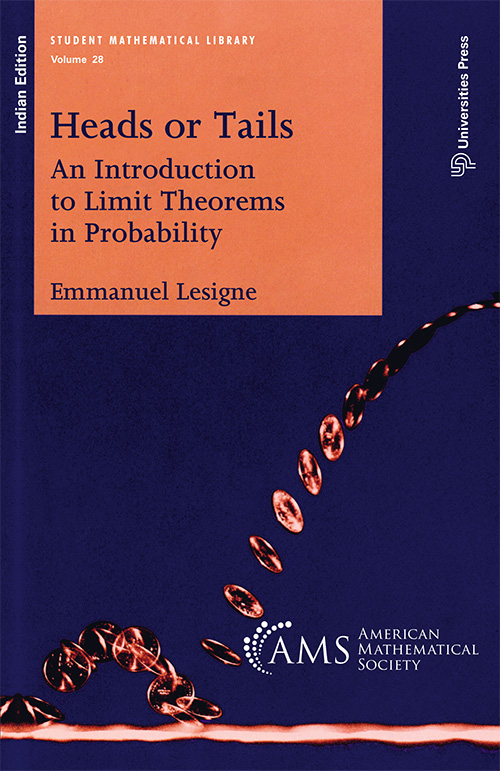## Heads or Tails: An Introduction to Limit Theorems in Probability

#### Price: 550.00

Everyone knows some of the basics of probability, perhaps enough to play cards. Beyond the introductory ideas, there are many wonderful results that are unfamiliar to the layman, but which are well within our grasp to understand and appreciate. Some of the most remarkable results in probability are those that are related to limit theorems—statements about what happens when the trial is repeated many times. The most famous of these is the Law of Large Numbers, which mathematicians, engineers, economists, and many others use every day.

In this book, Lesigne has made these limit theorems accessible by stating everything in terms of a game of tossing of a coin: heads or tails. In this way, the analysis becomes much clearer, helping establish the reader's intuition about probability. Moreover, very little generality is lost, as many situations can be modelled from combinations of coin tosses.

#### Contributors (Author(s), Editor(s), Translator(s), Illustrator(s) etc.)

Emmanuel Lesigne, Université François Rabelais, Tours, France

• Preface
• Prerequisites and Overview
• Chapter 1. Modeling a Probabilistic Experiment
• §1.1. Elementary Experiments
• §1.2. Sequences of Elementary Experiments
• Chapter 2. Random Variables
• Chapter 3. Independence
• Chapter 4. The Binomial Distribution
• Chapter 5. The Weak Law of Large Numbers
• Chapter 6. The Large Deviations Estimate
• Chapter 7. The Central Limit Theorem
• §7.1. Statement of the Theorem
• §7.2. Remarks
• §7.3. Applications
• §7.4. Proof of the Theorem
• Chapter 8. The Moderate Deviations Estimate
• Chapter 9. The Local Limit Theorem
• Chapter 10. The Arcsine Law
• §10.1. Introduction
• §10.2. Statement of the Theorems
• §10.3. The Reflection Principle
• §10.4. Proof of the Arcsine Law
• §10.5. Proof of the Law of Returns to the Origin
• Chapter 11. The Strong Law of Large Numbers
• §11.1. Almost Sure Events, Independent Events
• §11.2. Borel´s Strong Law of Large Numbers
• §11.3. Random Sequences Taking Several Values
• §11.4. Normal Numbers
• §11.5. The Borel-Cantelli Lemmas
• Chapter 12. The Law of the Iterated Logarithm
• §12.1. Introduction
• §12.2. Hausdorff´s Estimate
• §12.3. Hardy and Littlewood´s Estimate
• §12.4. Khinchin´s Law of the Iterated Logarithm
• Chapter 13. Recurrence of Random Walks
• §13.1. Introduction and Definitions
• §13.2. Nearest Neighbor Random Walks on Z
• §13.3. General Results about Random Walks
• §13.4. Recurrence of Random Walks on ZN
• Chapter 14. Epilogue
• §14.1. A Few More General Results
• §14.2. Closing Remarks
• Biographies
• Bibliography
• Index

`